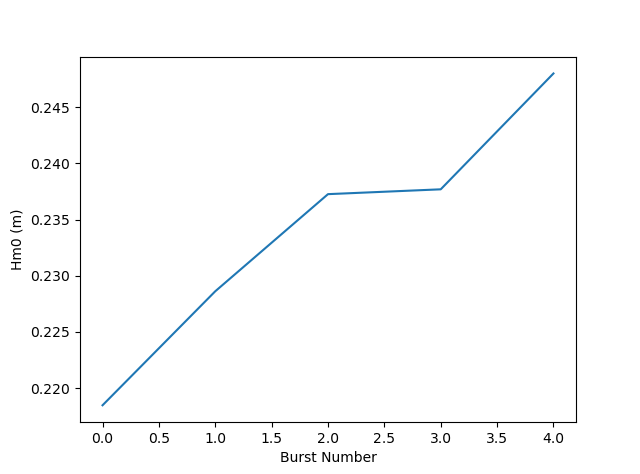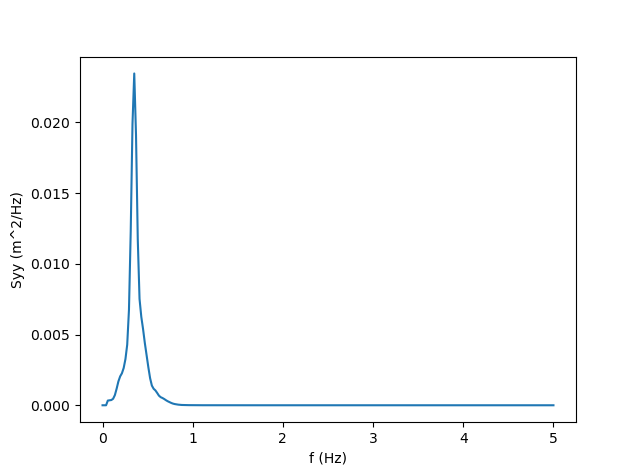# Tutorial (Python)#

Here is an example shows how to use OCEANLYZ. In this example, we use a provided sample file “waterpressure_5burst.csv” as input data. This sample file contains five bursts of water pressure data recorded with a pressure sensor. Sample file may be downloaded at akarimp/oceanlyz .

Measurement properties for “waterpressure_5burst.csv” are:

Properties

Value

OCEANLYZ Properties

File name

waterpressure_5burst.csv

Data type

Water pressure (Pa)

obj.InputType=’pressure’

Number of recorded burst (n_burst)

5

obj.n_burst=5

Sampling frequency (fs)

10 (Hz)

obj.fs=10

Recording duration (burst_duration)

1024 (second)

obj.burst_duration=1024

Pressure sensor height from bed (heightfrombed)

0.05 (m)

obj.heightfrombed=0.05

Mean water depth (h)

Varies in each burst

To start using OCEANLYZ, first, we need to be import required libraries.

#Import libraries
import oceanlyz
import os
import numpy as np
import matplotlib.pyplot as plt


Next, we download water pressure dataset (“waterpressure_5burst.csv”), we unzip it and copy sample files in a desired folder.

os.chdir('C:\\oceanlyz_python\\Sample_Data') #Change current folder to a folder that contains data file


We can plot data if we need to as:

plt.plot(water_pressure)
plt.xlabel('Sample points')
plt.ylabel('Water Pressure (N/m^2)')


Then, we need to create an OCEANLYZ object as:

#Create OCEANLYZ object
ocn = oceanlyz.oceanlyz()


Next, we assign wave data to OCEANLYZ object as:

#Input data
ocn.data = water_pressure.copy()


Now, we set up OCEANLYZ properties as:

ocn.InputType='pressure'
ocn.OutputType='wave+waterlevel'
ocn.AnalysisMethod='spectral'
ocn.n_burst=5
ocn.burst_duration=1024
ocn.fs=10
ocn.fmin=0.05
ocn.fmax=ocn.fs/2
ocn.fmaxpcorrCalcMethod='auto'   #Only required if ocn.InputType='pressure'
ocn.Kpafterfmaxpcorr='constant'  #Only required if ocn.InputType='pressure'
ocn.fminpcorr=0.15               #Only required if ocn.InputType='pressure'
ocn.fmaxpcorr=0.55               #Only required if ocn.InputType='pressure'
ocn.heightfrombed=0.05           #Only required if ocn.InputType='pressure'
ocn.dispout='yes'
ocn.Rho=1024                     #Seawater density (Varies)


After all required properties are set, we can run OCEANLYZ as:

ocn.runoceanlyz()


Output is stored as a structure array. Name of output is ‘oceanlyz_object.wave’. Field(s) in this structure array can be called by using ‘.’ For example oceanlyz_object.wave.Hm0 contains zero-moment wave height and oceanlyz_object.wave.Tp contains peak wave period.

Here we show how to plot zero-moment wave height:

Hm0 = ocn.wave['Hm0'] #zero-moment wave height
plt.plot(Hm0)
plt.xlabel('Burst Number')
plt.ylabel('Hm0 (m)')Figure 2: Plot of $$H_{m0}$$ versus burst number#

Similarly, we can plot wave spectrum for the first burst:

f = ocn.wave['f'] #frequency of the first burst
Syy = ocn.wave['Syy'] #spectrum of the first burst
plt.plot(f[0,:],Syy[0,:])
plt.xlabel('f (Hz)')
plt.ylabel('Syy (m^2/Hz)')Figure 3: Plot of $$S_{yy}$$ versus f#